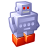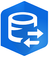# Calculating inter-point bearing between consecutive points?

2063
5
09-20-2016 11:16 AMNew Contributor II

Hi,

I have been pointed to a script for calculating the distance between consecutive points and now I need a script or some other way for calculating the bearing (N, NE, NW, etc) between the same points.

Bob

Tags (4)
5 RepliesbyEsri Regular Contributor

Here is a function:

# North azimuth bearing of a line segment (degrees)
def NorthAzimuth(x1,y1,x2,y2):
degBearing = math.degrees(math.atan2((x2 - x1),(y2 - y1)))
if (degBearing < 0):
degBearing += 360.0
return degBearingNew Contributor II

Bruce,

I have calculated azimuths already, what I need now is a script for

converting the numbers to text--N, NE, NW etc. I can't seem to find just

what I want so far. I've been looking at if/then statements in both VB and

Python.

Thanks,

BobbyMVP Esteemed Contributor

there are two different field calculator expressions in this link

``````"""  azimuth_to(shape, from_x, from_y)
input:      shape field, from_x, from_y
returns:    angle between 0 and <360 between a specified point and others
expression: azimuth_to(!Shape!, from_x, from_y)
"""
def azimuth_to(shape, from_x, from_y):
radian = math.atan((shape.centroid.X - from_x)/(shape.centroid.Y - from_y))
if degrees < 0:
return degrees + 360.0
else:
return degrees‍‍‍‍‍‍‍‍‍‍‍‍‍‍‍‍‍‍‍‍‍‍‍‍‍‍‍‍‍‍‍‍‍‍‍‍‍‍‍‍‍‍‍‍‍‍‍‍``````

Expression

``azimuth_to(!Shape!,from_x, from_y)‍‍‍‍``

Or this one

``````"""  angle_between(shape)
input:      shape field
returns:    angle between successive points,
NE +ve 0 to 90, NW +ve 90 to 180,
SE -ve <0 to -90, SW -ve <-90 to -180
expression: angle_between(!Shape!)
"""
x0 = 0.0
y0 = 0.0
angle = 0.0
def angle_between(shape):
global x0
global y0
x = shape.centroid.X
y = shape.centroid.Y
if x0 == 0.0 and y0 == 0.0:
x0 = x
y0 = y
return 0.0
radian = math.atan2((shape.centroid.Y - y0),(shape.centroid.X - x0))
x0 = x
y0 = y
return angle
‍‍‍‍‍‍‍‍‍‍‍‍‍‍‍‍‍‍‍‍‍‍‍‍``````

Expression

``angle_between(!Shape!)‍``

You can mess around with them to get what you want in terms in terms of inputs and outputs.New Contributor II

Dan,

With the scripts you sent earlier I was able to calculate azimuths. What I

need now is a script for converting the numbers to N, NE, NW etc. I can't

seem to find just what I want so far. I've been looking at if/then

statements in both VB and Python.

Thanks,

Bob

On Tue, Sep 20, 2016 at 8:19 PM, Chris Donohue, GISP <geonet@esri.com>byEsri Regular Contributor

Hi Bob

If you have your angles then you need to test they are within +/- 11.25 degrees of these azimuth breaks:

N:0

NNE:22.5

NE:45

ENE:67.5

... and so on.

BTW, the values are easy to calculate (obviously) but in a shameless plug for Esri's geocoding open file:///C:/Program%20Files%20(x86)/ArcGIS/Desktop10.4/Locators/USAddress.lot.xml in Firefox and navigate to the Spatial Operators elements - very few people know you can prepend spatial operators to street addresses and geocode "off road".